# Snubber Circuit Design Analysis

## What is a Snubber?

Snubber is a form of circuit protection against voltage spikes, ringing and oscillation effects. Snubber works by either clamping voltage spikes but not altering the ringing frequency or does the same function. Snubber circuit design is one of the complex tasks in circuit design. It needs a deep knowledge on circuit’s foundations to design a good snubber circuit. However, after reading this, you can design your own snubber circuit.

## General Classifications of Snubber

### Lossy or Dissipative

Lossy snubber circuit is the one that draws or consume power. In terms of system efficiency, using this is a disadvantage especially to power supplies that aims for very high efficiency requirement. However, this is less complex and easier to design. Dissipative snubber uses resistor and sometimes diode as dissipating elements.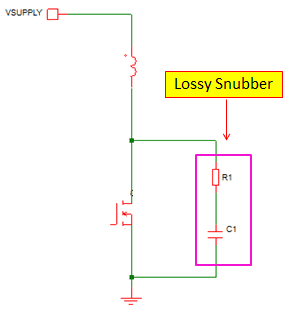### Non-lossy or Non-dissipative

Non-lossy snubber circuit is the one that will not consume power. This is a complex solution most of the times and also expensive. This is preferred for high efficiency application. Non-dissipative snubber uses inductors and capacitors.

### Lossy and Non-lossy Snubber Power Loss Comparison

Lossy Snubber losses depend on the selection of the snubber devices. The device selection is dependent to the level of voltage spike to suppress and the ringing frequency. For most applications, dissipative snubber losses are minimized and tolerable thus designers always use it for quick and easy design life.

Non-lossy snubber is ideally lossless or will not consume power. However, there is no such thing as ideal so it has small losses on it. In the past several snubber circuit design I did (speaking of a dissipative snubber), I manage to set the power loss to less than 1W for a 500W or even higher power. This corresponds to 0.2% of the output power which is negligible for the efficiency.

## Commonly Used Snubbers in Practical Applications

Despite of many snubber variations, snubber circuit design is often times concentrate only into two commonly used configurations.

### 1. RC SnubberFrom the name itself, it is uses resistor and capacitor to form a snubber circuit. This is commonly used snubbers for switching MOSFETs.

#### Some Circuits Where RC Snubber Circuit Used

Boost Converter TopologyBuck ConverterDCDC Synchronous Rectifier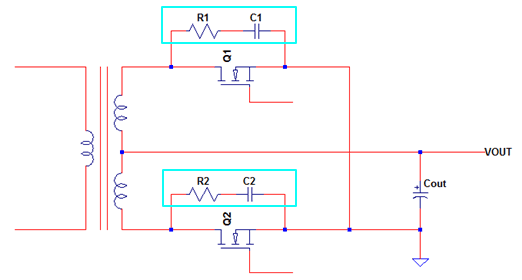### 2. RCD Snubber

For some designers, they called this as RCD clamp more often that RCD snubber.

It is because it will literally clamp the voltage spikes while not modifying the spike or ringing frequency. RCD snubber is consisting of resistor, capacitor and diode.#### Some Circuits Where RCD Snubber is used

Flyback ConverterForward Converter## How RC Snubber Works

RC snubber is commonly used in switching converters to limit the voltage spike on the switching device into a safe level. Not just by simply clamping the voltage spike alone, but also modifying the spike frequency or ringing behavior to prevent further issue.RC snubber works by modifying the ringing frequency as well as lowering the voltage spike level. The capacitor acts as charge storage and the resistor provides a discharge path. For instance in below circuit, the RC snubber R1 and C1 protects the MOSFET Q1 from voltage spike on the drain. When the MOSFET is OFF, the snubber capacitor will charge through R1. When the MOSFET turns ON, the capacitor will discharge through R1 to the MOSFET and to the circuit ground. The cycle will repeat with the capacitor is empty. The resistor is the one dissipates power. In a single switching cycle, there are two times where current flows to the resistor. Below illustration called the currents as charge and discharge currents.The fact that an RC snubber is capable of modifying the ringing frequency, it is instrumental in solving EMI related issues. In the past, I had solved several issues in EMI b using RC snubbers on switching MOSFET and diodes.

## What Creates Ringing and Voltage Spikes in Switching MOSFET?

Ringing and voltage spike is caused by the interaction of leakage inductance and MOSFET output capacitance. It is the leakage inductance that will give voltage spike and not the desired inductance. The leakage inductance will store energy but this energy is not being transferred to the load or to a desired system and thus nowhere to go.

Below diagram is a common synchronous rectifier in center tap full bridge rectification. This circuit structure is common in DCDC section of an SMPS. The energy in the desired inductance will be transferred to the load (output side) but the leakage energy has nowhere to go.Q1 and Q2 in the above diagram will not operate in the same time. When Q1 is ON, Q2 is OFF and vice versa. The circuit is can be simplified by taking only individual MOSFET as below.The VDD level is just ideally twice the level of the output plus the spike.

## RC Snubber Circuit Design and Analysis

There is no perfect method to use in selecting RC snubber components. However, there are techniques that are proven working well in actual design. One of the documents that I also refer to is the application note of NXP semiconductor, AN11160 which is entitled Designing RC snubbers. https://www.mikrocontroller.net/attachment/202527/AN11160_Designing_RC_Snubbers__NXP.pdf This is a good theoretical and practical approach to select RC snubbers starting values.

The critical parameters to consider for the selected parts are voltage and power dissipation of the snubber resistor. The voltage rating of the capacitor and resistor must be higher than the voltage spike.

## Power Loss in RC Snubber

The contributor of power loss in RC snubber is the resistor. The right resistor size must be selected in terms of power loss and snubber effectiveness. Too high resistance has lower power loss but might deliver not effective snubber. On the other hand, lower resistance most likely can deliver an effective snubber but the efficiency of the system will suffer due to higher power loss in RC snubber.

### How to Calculate Power Loss in RC Snubber Resistor

In below circuit, Rsn and Csn comprise the RC snubber network. When Q1 is turning on, the charge on the snubber capacitor will pass through Rsn to discharge. By the moment Q1 turns off, the capacitor Csn will charge through Rsn. Therefore, in a single switching cycle, the current will pass through the resistor twice.Below are the important waveforms for the analysis. The total RMS power dissipation on the resistor is dependent to the VRMS1 and VRMS2. Actually, the RMS1 waveform is on the negative y-axis because it is happening when the capacitor discharges. However, since we are going to get the RMS value we draw the waveform in the positive y-axis. For RMS derivation, it will not matter.Definitions:

VRMS1 – RMS value of the resistor voltage waveform when the capacitor is discharging

VRMS2 – RMS value of the resistor voltage waveform when the capacitor is charging

VDRAIN – Drain voltage of Q1

VCSN – Snubber capacitor voltage

VRSN – Snubber resistor voltage

PWM – pulse width modulated signal on the gate of Q1 to turn it on and off

T – One switching period

Ton – the time that Q1 is on or the PWM is high

5RC – simply 5 tau or 5 time constants

t1 – the time the voltage on the resistor become zero after Q1 turns off

Note: on below derivations, the area under the curve is considered as triangular for easier integration. Thus the computed result is may be a bit higher than the actual test result.

Derivations:

t1

When Q1 is off, the snubber capacitor will charge and its voltage will rise exponentially while the snubber resistor will see very high voltage initially but decay exponentially, ThusWhere;

VDS – steady state of the drain voltage of Q1 (without the spike)

VDSMAX – is the peak drain voltage (with the spike)

VRMS1

VRMS1 is present from time zero to full discharge state of the capacitor which happening at 5 time constants.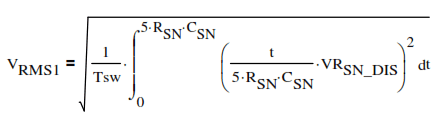Where;

VRSN_DIS – peak level of the resistor voltage during discharge. This is equal to the level of the drain voltage without the spike.

VRMS2

VRMS2 is present from the moment Q1 is off until t1. So,Where;

VRSN_CHA – peak level of the resistor voltage during charging (with voltage spike)

Total RMS Voltage of Snubber Resistor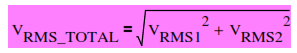Snubber Resistor Power Dissipation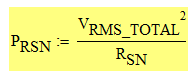Example Power Loss in RC Snubber Calculation

This design example features a center tap transformer with a synchronous rectifier on each transformer leg. Q1 and Q2 have complementary operations (ideally 50% duty not considering dead time).

When Q1 is off, its drain voltage will see initially a high voltage spike then settle to twice the Vout level since L1 and L2 has the same number of turns. During this time C1 will charge.

When Q1 turns on, it will provide ground path for the charge in C1 to discharge through R1. By this time, the peak level of the voltage on R1 is just twice Vout plus the drop on Q2.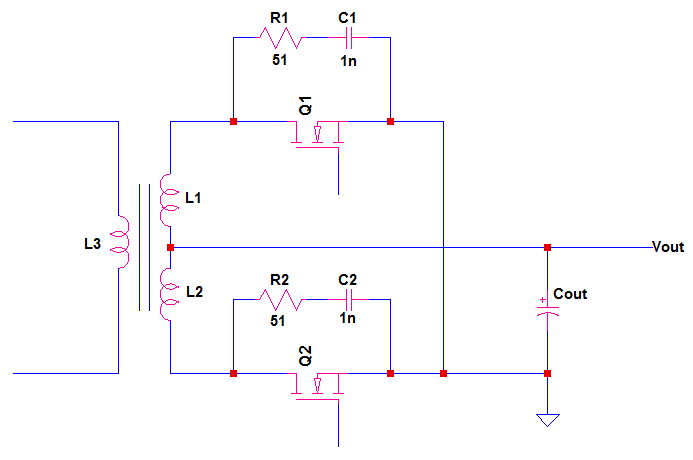Given:

Vout = 24V

CSN = 1nF

RSN = 51Ω

Fsw = 110kHz (switching frequency)

VDSMAX = 80V (measured voltage spike)

VSR_DROP = 0.2V (estimated voltage drop of Q1 or Q2 when on)

Solution: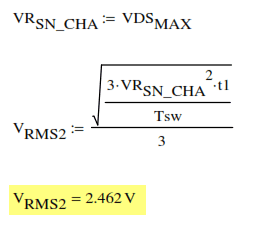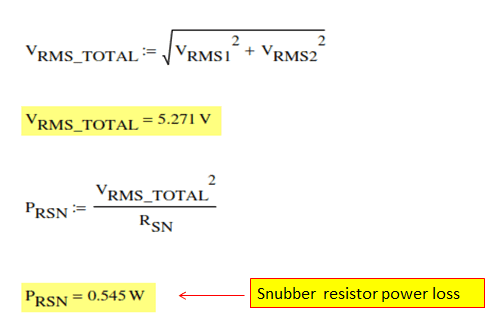## Short Cut Method to Compute Power Loss in RC Snubber

Above solution is complicated and need very high technical knowledge. There is a straight forward solution that can be used. This is good enough if you have big margin on the design since this will give higher power dissipation. If you want a more realistic result however, you must use the previous analysis.

In this approach, the energy in resistor is equated to the capacitor in both charge and discharge state. I can agree for the discharge state since it is obvious that the energy in the capacitor is nowhere to run than in the resistor not considering the small damping effect of the MOSFET on state resistance. However, I cannot agree to this during the time that the capacitor is on the act of charging. Anyway, just to demonstrate this approach, let us buy the idea that the energy during charging and discharging of the capacitor is the same and this will also the energy on the resistor.Since the energy during charging and discharging have the same magnitude, we can only consider either one of them to get the energy. Let us consider the discharge state in this analysis.

So,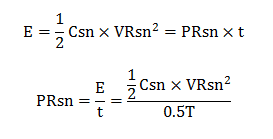Considering a switching frequency of 110 kHz and a maximum drain voltage of 80V while 1nF for the snubber capacitor, the resulting power dissipation on the resistor is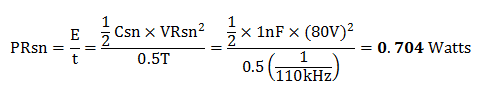The result on this approach is higher than the previous method and the actual or measured result. The previous method (the long one) recorded 0.545 watts; while this short cut method result is 0.704 watts.

## RCD Snubber Circuit Design and Analysis

RCD snubber is sometimes called RCD clamp as it actually clamps the voltage spikes. RCD clamps acts as low impedance voltage source. It has three main components as the name implies; resistor for R, capacitance for C D for diode. The resistor will dissipate power from the stored leakage energy while the capacitor ensures a low ripple DC source. The diode acts as a unidirectional switch. Below circuit enclosed in blue rectangle is the RCD snubber.

RCD clamp or snubber is commonly used in flyback converter, so we will design RCD values based from flyback.In order to derive the equations, it is very important to know the waveform and how to analyze it. I am showing here the derivations but do not mind to memorize it, just use the formula I already derived.

### Derivation of Rsn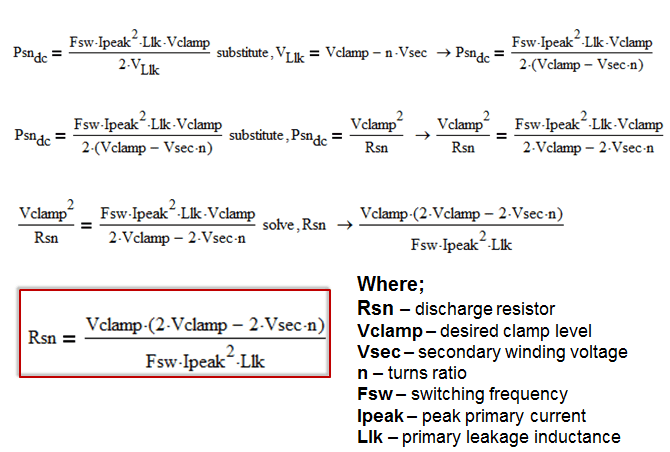In the above equation, “OF” is short for “other factor”. In the previous derivations, it is assumed that the current will only flow to the RCD clamp and that diode Dsn has ideally zero forward recovery time. In actual design, portion of the current may flow to the drain capacitor and the forward recovery time of the diode is not zero. These will make the computed clamp voltage smaller than the actual. To compensate this, additional margin must be added. A good estimate is to set OF to 20-30%.

### Derivation of Csn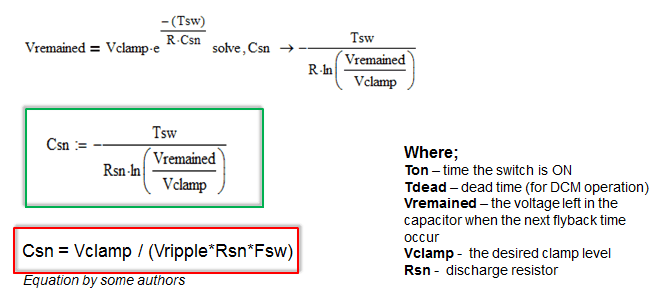• Several authors said that the capacitor must be large enough so that the clamp voltage will not vary a lot in one switching cycle.
• A good starting point in choosing the capacitor is to set the ratio of Vremained to Vclamp to 50%. This means that the ripple voltage of the clamp network is half of the clamp voltage.

The power dissipation on the resistor is can be computed as:

PRsn = Vclamp2 / Rsn

1. Ahmed Helmy says:

The most wonderful explanation I’ve ever seen,Thanks alot

1. tolga says:

2. Soumya Chatterjee says:

I really want to appreciate your Efforts.
You have presented in a very good manner.

1. electronicsbeliever says:

This may be late, but thank you for the compliment.

3. Kevin says:

What will cause if RCD snubber’s Csn choose too large?

4. dothuy van says:

good

5. There are wrong in the dot of transformer of forward converter in Some Circuits Where RCD Snubber is used

1. electronicsbeliever says:

thanks Tahar, good catch…dots must be on same side.

6. lan says:

“The VDD level is just ideally twice the level of the output plus the spike.”
Ok but the circuit shows nothing labeled output! Rather confusing.

7. Vicarzo says:

I really thank you very much, for this very detailed explanation.

1. electronicsbeliever says:

Thank you!

8. Siddharth Saxena says:

why do we use snubber capacitance if resistance can dessipate the energy stored due to leakage inductance .

1. electronicsbeliever says:

This is a good question.
If only resistor is installed, it will conduct all the time. It will interfere to the normal operation of the switching converter. Moreover, the resistor will burn due to huge rms power.
The capacitor will only allow ac current in a short duration. Once it is full, it will act as open circuit and there is no more power dissipation on the resistor.